Ex 8.1

Chapter 8 Class 6 Decimals
Serial order wise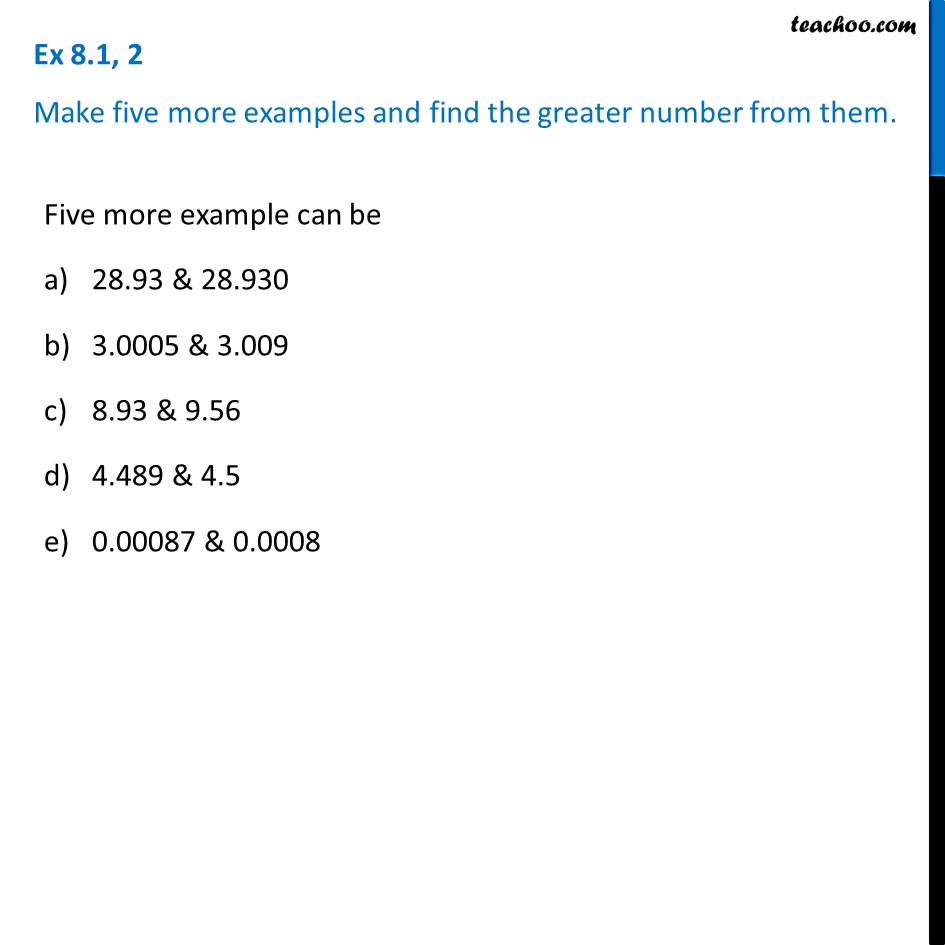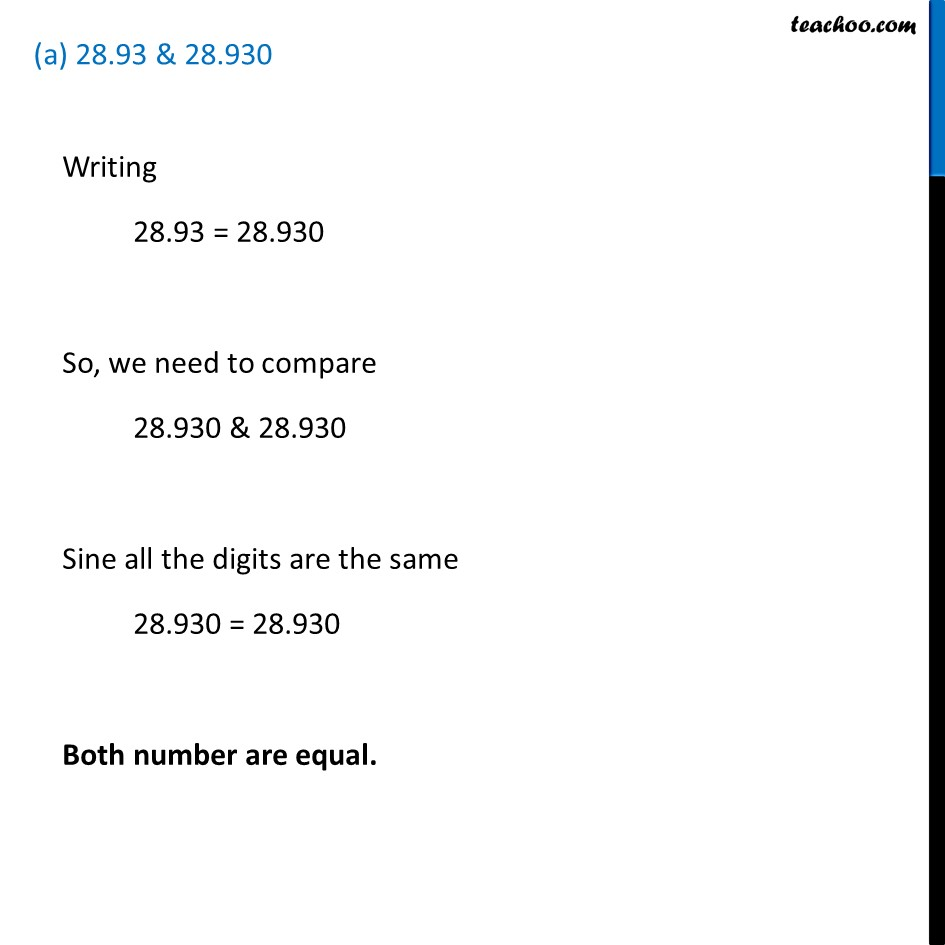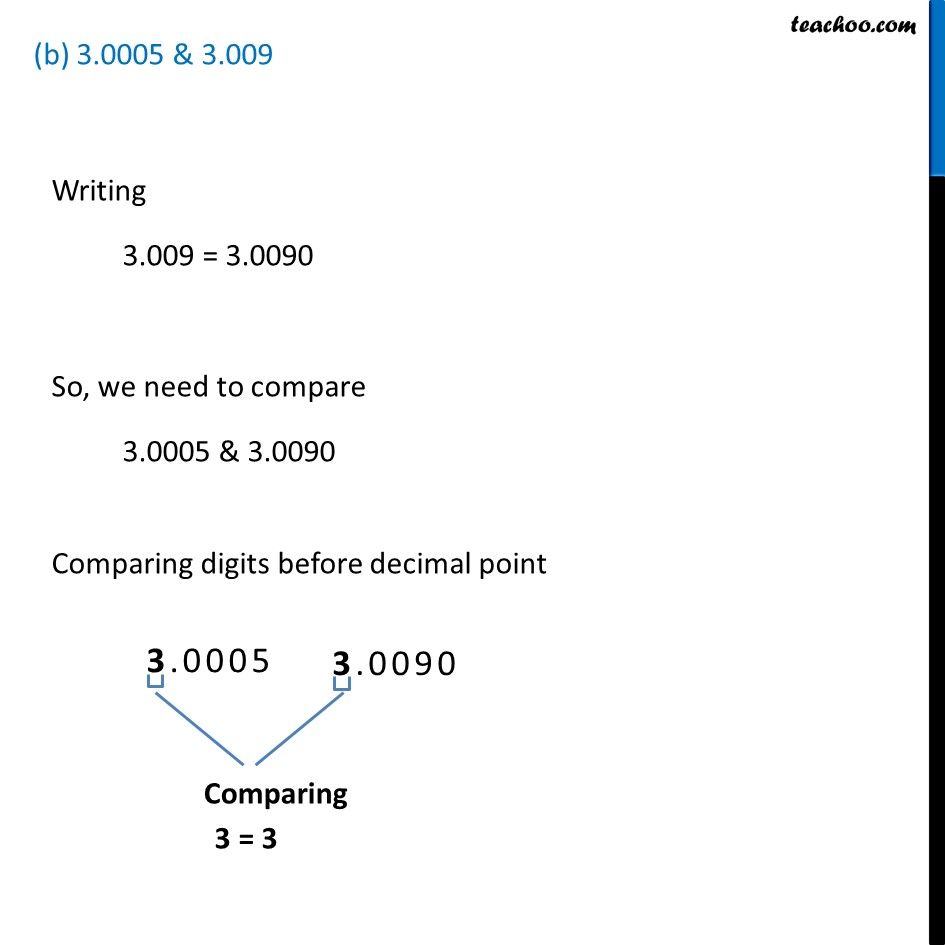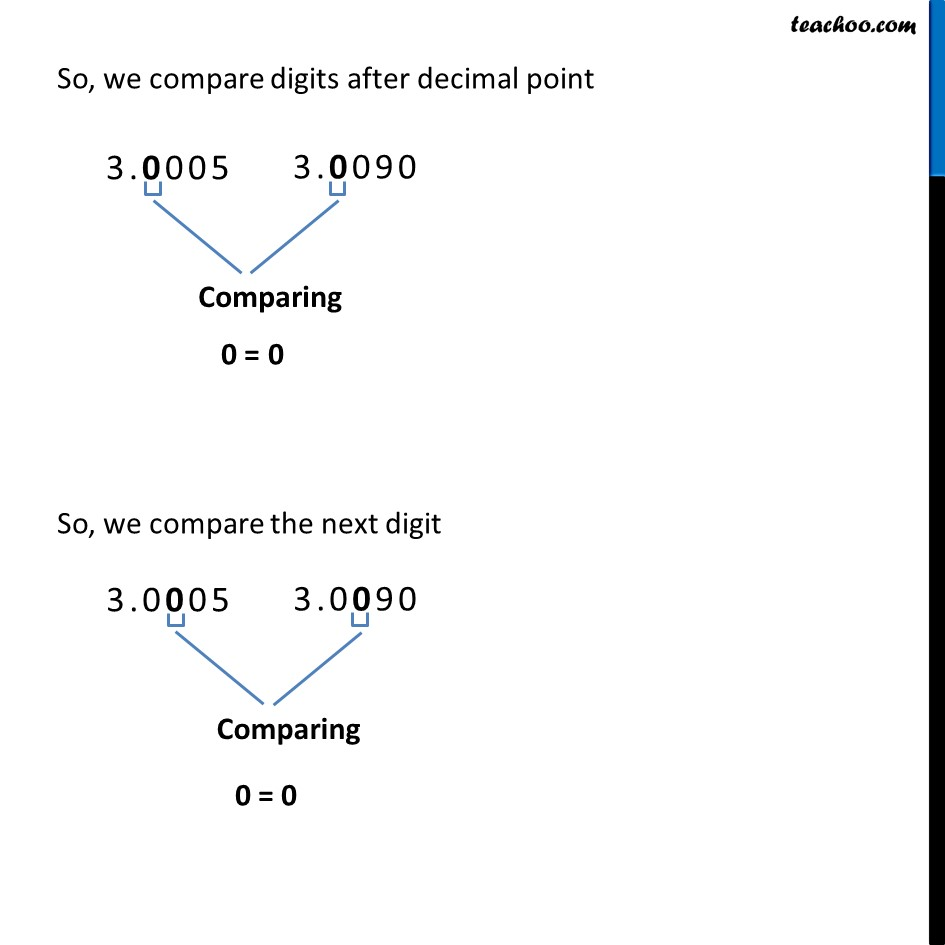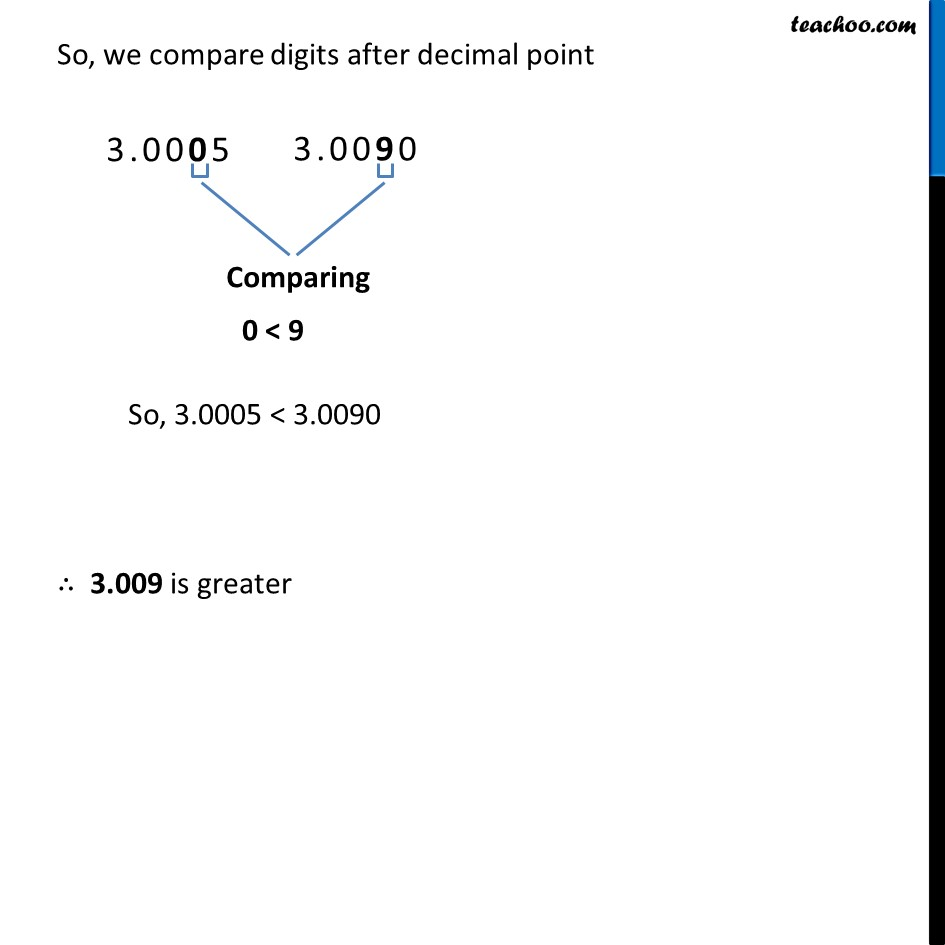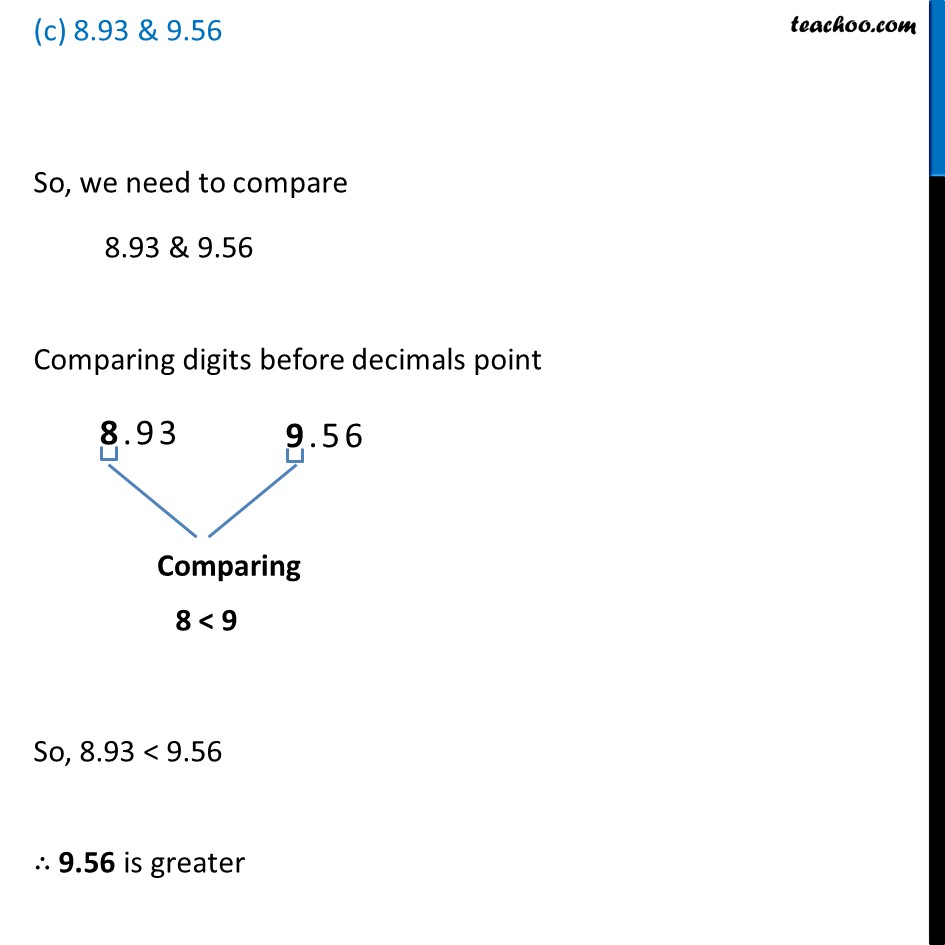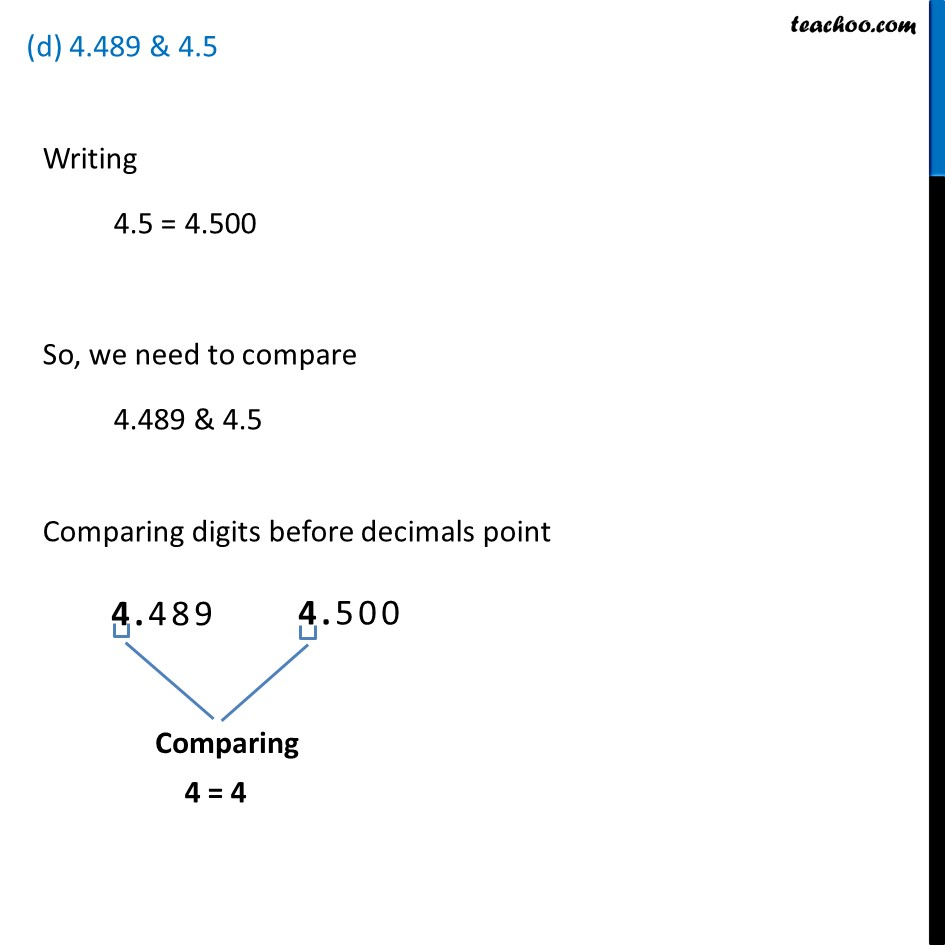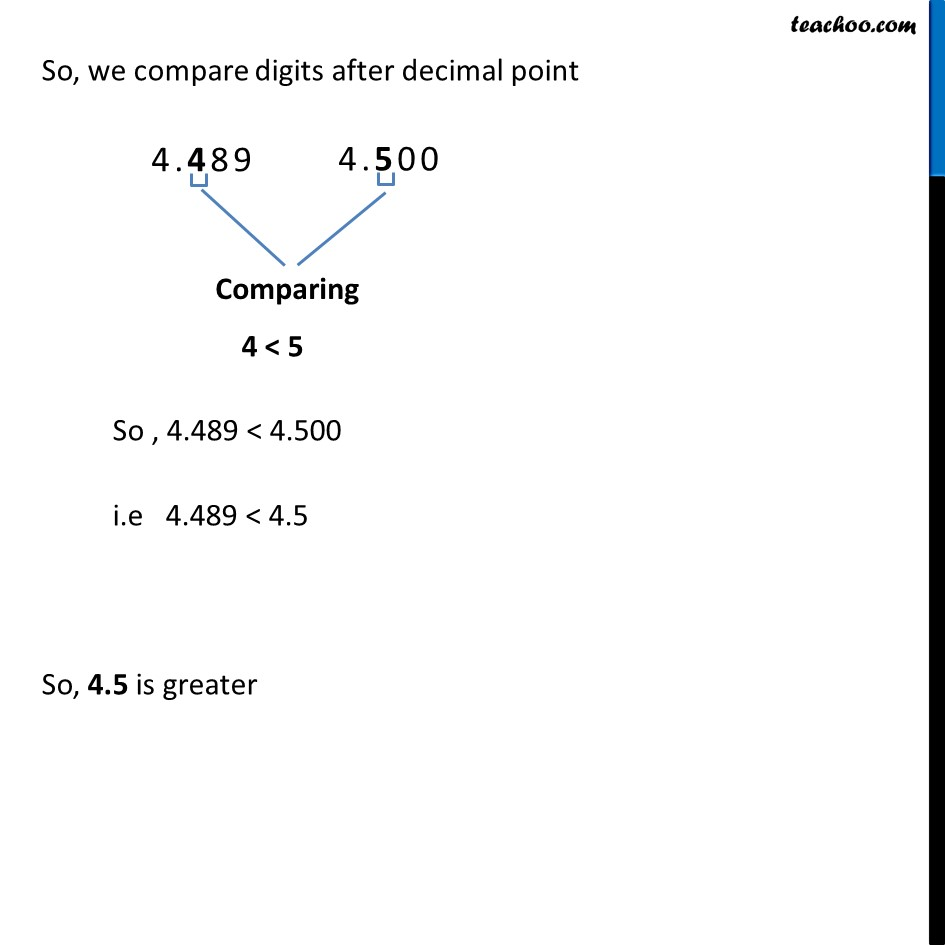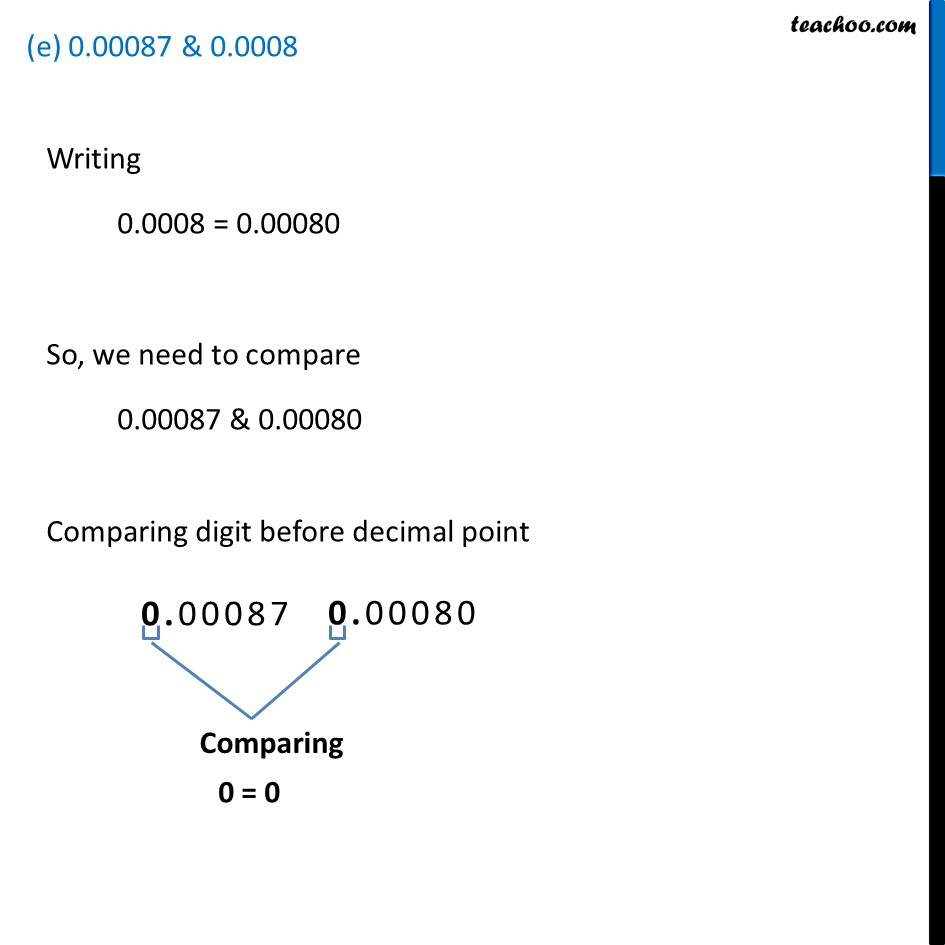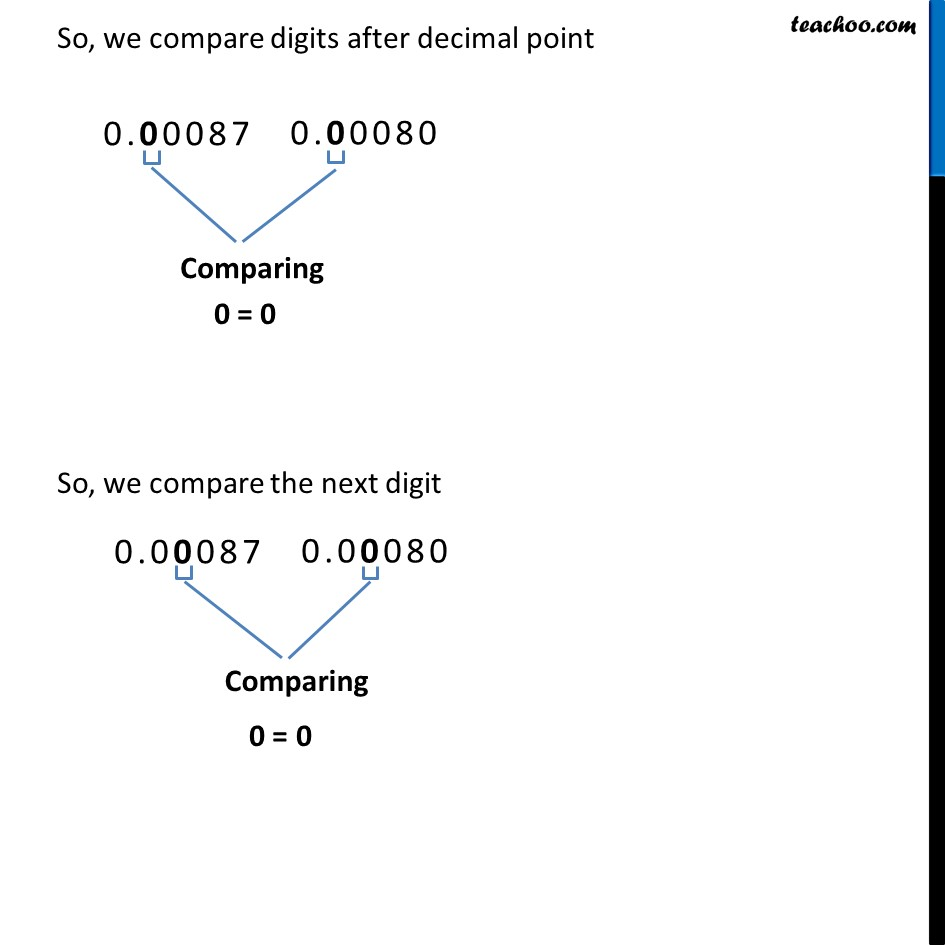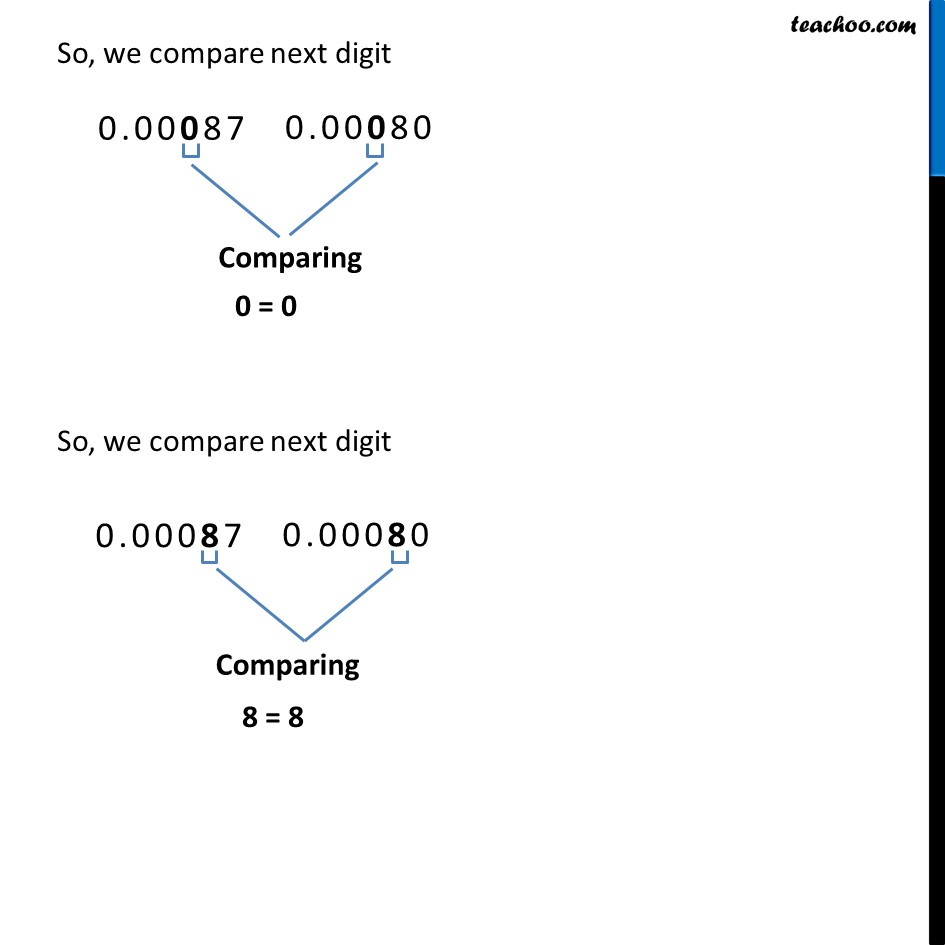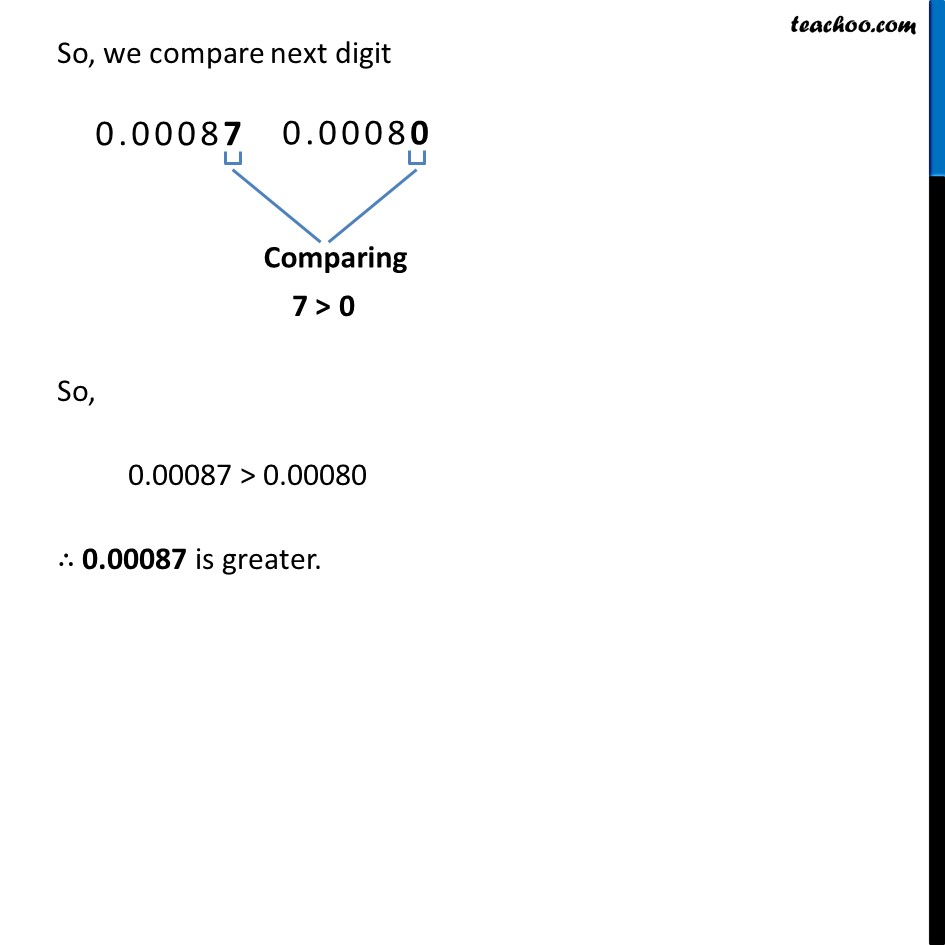Learn in your speed, with individual attention - Teachoo Maths 1-on-1 Class

### Transcript

Ex 8.1, 2 Make five more examples and find the greater number from them. Five more example can be 28.93 & 28.930 3.0005 & 3.009 8.93 & 9.56 4.489 & 4.5 0.00087 & 0.0008 (a) 28.93 & 28.930Writing 28.93 = 28.930 So, we need to compare 28.930 & 28.930 Sine all the digits are the same 28.930 = 28.930 Both number are equal. (b) 3.0005 & 3.009Writing 3.009 = 3.0090 So, we need to compare 3.0005 & 3.0090 Comparing digits before decimal point 3 = 3 So, we compare digits after decimal point 0 = 0 So, we compare the next digit 0 = 0 So, we compare digits after decimal point 0 < 9 So, 3.0005 < 3.0090 ∴ 3.009 is greater (c) 8.93 & 9.56So, we need to compare 8.93 & 9.56 Comparing digits before decimals point 8 < 9 So, 8.93 < 9.56 ∴ 9.56 is greater (d) 4.489 & 4.5Writing 4.5 = 4.500 So, we need to compare 4.489 & 4.5 Comparing digits before decimals point 4 = 4 So, we compare digits after decimal point 4 < 5 So , 4.489 < 4.500 i.e 4.489 < 4.5 So, 4.5 is greater (e) 0.00087 & 0.0008Writing 0.0008 = 0.00080 So, we need to compare 0.00087 & 0.00080 Comparing digit before decimal point 0 = 0 So, we compare digits after decimal point 0 = 0 So, we compare the next digit 0 = 0 So, we compare next digit 0 = 0 So, we compare next digit 8 = 8 So, we compare next digit 7 > 0 So, 0.00087 > 0.00080 ∴ 0.00087 is greater.